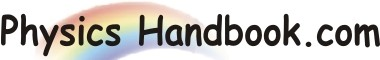HOME TOPICS DEFINITIONS TABLES LAWS INVENTIONS EXPERIMENTS QUIZ VIDEOS
 A B C D E F G H I J K L M N O P Q R S T U V W X Y Z
Electromotive Force

If we want that electric current should flow through a conductor, we must maintain potationtial difference (p.d) across its ends. A device that maintains potential difference between two points is said to develop and electromotive force(e.m.f.).

The simple example of such device is that of a cell consists of two dissimilar metal plates(say copper & zinc) immersed in an electrolyte (say dil. H 2SO4)as shown in fig (a). The chemical action in the cell cause copper plate to become positively charged and zinc plate negatively charged, causing a potential difference to exist between the terminals of cell. The maximum p.d.(e) between the two plates of a cell on open-circuit(i.e. cell delivering no current )is called electromotive force (e.m.f) of the cell.(see the fig (a))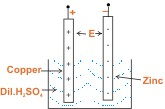Fig (a)

If the two plates are joined through a wire as shown in fig (b) ,an electron is attracted from zinc plate through the wire to copper plate .the charges on the plates are reduced by one electron. The chemical action of the cell now transfer one electron from copper plate to zinc plate internally through the cell . Thus, plates acquire original charge to maintain original p.d. this process goes on so long as the circuit is complete or so long as there is chemical energy available.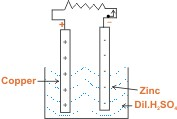Fig (b)
The e.m.f. of a cell can also be define as the energy supplied by the cell to drive a unit charge once around the complete circuit and is given by;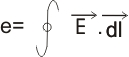where,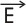= Electric field i.e. Force per unit charge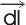= Small part Segment Vector

Since, e.m.f. of a cell is the potential difference between its plates on open circuit ,one can expect that s1 unit of e.m.f. is volt.

Note:
Unfortunately,the early scientists called e.m.f. a force instead of incorporating the word work into the terminology.However, e.m.f. has unit of work and not unit of force.